# Anyone for a game of checkers?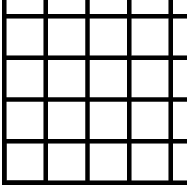Suppose you have a checkerboard that extends infinitely far above and to the right.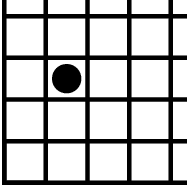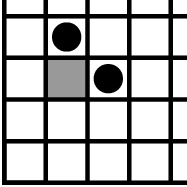You are not allowed to move checkers on the board.  However, you may remove a checker provided you replace it with two more, one above and one to the right of where the checker was removed.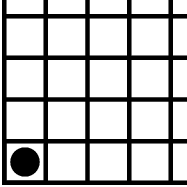Suppose that you begin with a single checker in the lower left corner.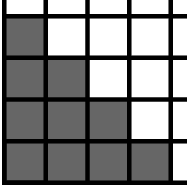Can you arrange the checkers, without stacking them, so that there are none in the shaded region?

Here is one way you may get started.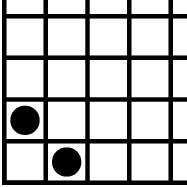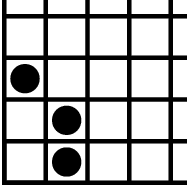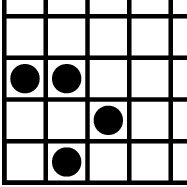There is no way to get the checkers out of the shaded region.  Here is one way to see this:

Assign numbers to each of the squares, beginning with 1 in the lower right square.  When you move up or to the right, the number in a square is divided by 2.

Now think about the sum of the numbers in the squares that contain checkers.  When there is a single checker in the lower left corner, this sum is 1.  However, when a checker is removed and replaced with two checkers, this sum will not change.  Therefore, the sum is always 1.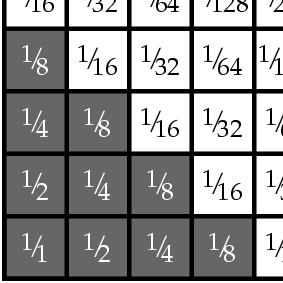Let’s determine the sum of the numbers in all the squares.  Notice that the numbers in the bottom row form a geometric series:

1 + ½ + 1/4+ 1/8 + 1/16 + … = 2.

Since the numbers in the second row are half those in the first, the sum of the numbers in that row is 1.  Likewise, the sum of numbers in the third row is ½.  Therefore, the sum of all the numbers is

2 + 1 + ½ + ¼+1/8+1/16+… = 4.

By subtracting the numbers in the shaded region, we can then determine that the sum of numbers in the unshaded region is ¾.

Since the sum of the numbers in squares containing a checker must be 1, there is no way to move all the checkers into the unshaded area.

This is a problem that Matthew Stamps encountered during the Budapest Semester in Mathematics.